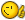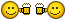# help! Masters; EXTRACT VERTEX X,Y choose first point at way clockwise (need upgrade)

## Recommended PostsI had this from Lee, but usually always go way counter-clockwise and only select the polyline, not the point.

;; Polyline Vertex Exporter ~ by Lee McDonnell ~ 26.11.2009

(defun c:pExp2 (/ ss tmp i j ent tot dis pt)

(if (and (setq ss (ssget '((0 . "*POLYLINE"))))

(setq tmp (getfiled "Output File" (cond (*load) ("")) "txt;csv" 9)))

(progn

(setq *load tmp tmp (open tmp "a") i -1)

(write-line "X,Y,Layer" tmp)

(while (setq ent (ssname ss (setq i (1+ i))))

(setq tot 0. j (1- (vlax-curve-getStartParam ent)))

(while (

(setq pt (mapcar 'rtos (vlax-curve-getPointatParam ent j)))

(write-line

(strcat (car pt) (chr 44) (cadr pt) (chr 44) (vla-get-layer (vlax-ename->vla-object ent)))

tmp))

(write-line "" tmp))

(close tmp)))

(princ))

THX for any tips.

##### Share on other sitesWow, that's some old code...

I don't quite understand, what do you want the code to do?

[highlight][noparse]

`[/noparse][/highlight]Your code here[highlight][noparse]`

[/noparse][/highlight]

##### Share on other sitesI´ll try explian;

the file returns the polyline reverse clockwise way sample;

X,Y,Layer

485172.23,6691696.77,FC 0059

485157.93,6691697.48,FC 0059

485156.58,6691666.50,FC 0059

485171.15,6691665.78,FC 0059

485172.23,6691696.77,FC 0059

I would like th clockwise side return, (above)

485172.23,6691696.77,FC 0059

485171.15,6691665.78,FC 0059

485156.58,6691666.50,FC 0059

485157.93,6691697.48,FC 0059

If can select the FIRST vertex it will be perfect;

take this oportunite to say I follow this years, your are the guy, congrates

Edited by leo321

##### Share on other sitesThank you for your compliments```(defun c:ptx ( / *error* des ent enx lay lst txt )

(defun *error* ( msg )
(if (= 'file (type des)) (close des))
(if (and msg (not (wcmatch (strcase msg t) "*break,*cancel*,*exit*")))
(princ (strcat "\nError: " msg))
)
(princ)
)

(if (setq txt (getfiled "Create Output File" (cond ( ptx:dir ) ( "" )) "txt;csv" 1))
(while
(not
(progn (setvar 'errno 0) (setq ent (car (entsel "\nSelect 2D polyline <Done>: ")))
(cond
(   (= 7 (getvar 'errno))
(prompt "\nMissed, try again.")
)
(   (null ent))
(   (/= "LWPOLYLINE" (cdr (assoc 0 (setq enx (entget ent)))))
(prompt "\nThe selected object is not a 2D polyline.")
)
(   (not
(or des
(and (setq des (open txt "w"))
(setq ptx:dir (strcat (vl-filename-directory txt) "\\"))
(write-line "X,Y,Layer" des)
)
)
)
(princ (strcat "\nUnable to open \"" txt "\" for writing."))
)
(   (setq lst (mapcar 'cdr (vl-remove-if-not '(lambda ( x ) (= 10 (car x))) enx))
lay (list "," (strcat "," (cdr (assoc 8 enx))))
)
(foreach vtx (if (LM:listclockwise-p lst) lst (reverse lst))
(write-line (apply 'strcat (mapcar 'strcat (mapcar 'rtos vtx) lay)) des)
)
(write-line "" des)
(prompt (strcat "\n" (itoa (length lst)) " vertices written to " (vl-filename-base txt) (vl-filename-extension txt) "."))
)
)
)
)
)
(princ "\n*Cancel*")
)
(*error* nil) (princ)
)

;; List Clockwise-p - Lee Mac
;; Returns T if the point list is clockwise oriented

(defun LM:listclockwise-p ( lst )
(minusp
(apply '+
(mapcar
(function
(lambda ( a b )
)
)
lst (cons (last lst) lst)
)
)
)
)

(princ)```

##### Share on other sitesAnd another, allowing optional selection of a start point:

```(defun c:ptx ( / *error* cnt des dis ent enx idx lay lst spt tmp txt )

(defun *error* ( msg )
(if (= 'file (type des)) (close des))
(if (and msg (not (wcmatch (strcase msg t) "*break,*cancel*,*exit*")))
(princ (strcat "\nError: " msg))
)
(princ)
)

(if (setq txt (getfiled "Create Output File" (cond ( ptx:dir ) ( "" )) "txt;csv" 1))
(while
(not
(progn (setvar 'errno 0) (setq ent (car (entsel "\nSelect 2D polyline <Done>: ")))
(cond
(   (= 7 (getvar 'errno))
(prompt "\nMissed, try again.")
)
(   (null ent))
(   (/= "LWPOLYLINE" (cdr (assoc 0 (setq enx (entget ent)))))
(prompt "\nThe selected object is not a 2D polyline.")
)
(   (not
(or des
(and (setq des (open txt "w"))
(setq ptx:dir (strcat (vl-filename-directory txt) "\\"))
(write-line "X,Y,Layer" des)
)
)
)
(princ (strcat "\nUnable to open \"" txt "\" for writing."))
)
(   (setq lst (mapcar 'cdr (vl-remove-if-not '(lambda ( x ) (= 10 (car x))) enx))
lst (if (LM:listclockwise-p lst) lst (reverse lst))
lay (list "," (strcat "," (cdr (assoc 8 enx))))
)
(if (setq spt (getpoint "\nSpecify start point <use first vertex>: "))
(progn
(setq idx 0
cnt 1
spt (trans spt 1 ent)
dis (distance spt (car lst))
)
(foreach pnt (cdr lst)
(if (< (setq tmp (distance spt pnt)) dis)
(setq dis tmp
idx cnt
)
)
(setq cnt (1+ cnt))
)
(repeat idx (setq lst (append (cdr lst) (list (car lst)))))
)
)
(foreach vtx lst
(write-line (apply 'strcat (mapcar 'strcat (mapcar 'rtos vtx) lay)) des)
)
(write-line "" des)
(prompt (strcat "\n" (itoa (length lst)) " vertices written to " (vl-filename-base txt) (vl-filename-extension txt) "."))
)
)
)
)
)
(princ "\n*Cancel*")
)
(*error* nil) (princ)
)

;; List Clockwise-p - Lee Mac
;; Returns T if the point list is clockwise oriented

(defun LM:listclockwise-p ( lst )
(minusp
(apply '+
(mapcar
(function
(lambda ( a b )
)
)
lst (cons (last lst) lst)
)
)
)
)

(princ)```

##### Share on other sitesThanks a lot, when come to Brazil your beer is guarantees, it´ll be a honor.

##### Share on other sites..

..## Join the conversation

You can post now and register later. If you have an account, sign in now to post with your account.
Note: Your post will require moderator approval before it will be visible.×   Pasted as rich text.   Paste as plain text instead

Only 75 emoji are allowed.